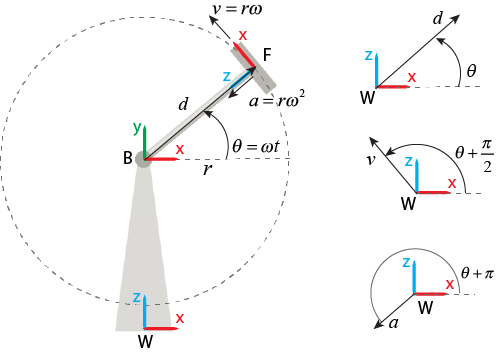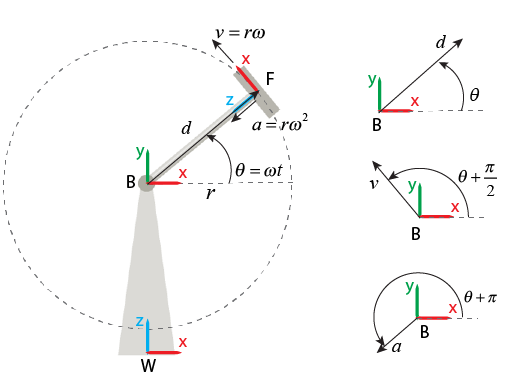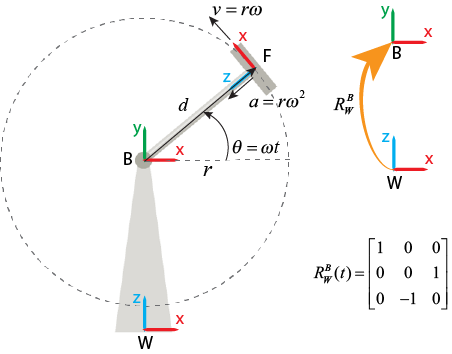## Selecting a Measurement Frame

You can use the Transform Sensor block to measure the relative relationship between two arbitrary frames that are connected to the B and F frame ports of the block. The relationship includes relative rotation, translation, and their first and second time derivatives. These measurements are 3-D vectors or higher dimensioned quantities, such as rotation matrices.

To do computation with the measured vectors, the vectors must be resolved in coordinates. The setting of Measurement Frame parameter determines where to resolve the measured vectors; the vectors are resolved in the selected frame's coordinates. For example, in the figure, because Measurement Frame was set to `World`, the Transform Sensor block resolves a translation vector, shown as a black arrow, in the world frame's coordinates.Note

The rotation measurement of the Transform Sensor block is independent of the Measurement Frame parameter.

### Measurement Frames

You can set the Measurement Frame parameter to `World`, `Base`, `Follower`, ```Non-Rotating Base```, or ```Non-Rotating Follower```.

#### World

The Transform Sensor block resolves and observes the measured vectors by using the world frame. For an example, see World.

The world frame is an inertial frame.

#### Base or Follower

The Transform Sensor block resolves and observes the measured vectors by using the selected base or follower frame.

The base or follower frame is the frame that connects to the block's B or F port, respectively. The base and follower frames are non-inertial. Therefore, the vectors resolved in the base or follower frame may involve centripetal and Coriolis terms.

#### Non-Rotating Base or Non-Rotating Follower

The Transform Sensor block maps the vectors resolved in the world frame to the selected frame that is non-rotating base or non-rotating follower frame. In other words, the block calculates the rotation matrix from the world frame to the current base or follower frame then multiplies the matrix with the vectors resolved in the world frame.

The non-rotating base or non-rotating follower frame is an instantaneous frame that is coincident and aligned with the corresponding base or follower frame at the current time. The measurements resolved in the non-rotating frames do not involve centripetal and Coriolis terms.

The table compares the properties of the measurements for the different Measurement Frame settings.

Measurement FrameStandard Derivative Relationship
WorldYes
BaseYes
FollowerYes
Non-Rotating BaseNo
Non-Rotating FollowerNo

When a selected frame satisfies the standard derivative relationship, the measurements resolved in this frame are related to each other. For example, when you select `World`, the resolved linear acceleration vector is the time derivative of the resolved linear velocity vector, which is the time derivative of the resolved linear translation vector.

### Example

This example shows the measurements of the Transform Sensor block with different settings of the Measurement Frame parameter. The image illustrates a single degree-of-freedom system with four parts: a support, hub, rod, and car. The support is fixed on the ground, and the rod connects the hub and car. The base, follower, and world frames of the system are located at the center of the hub, car, and support’s bottom, respectively. Note that the world and base frames are fixed frames and the follower frame moves with the car.The rod has a length of $r$ and rotates with a constant angular velocity, $\omega$, around the `Z`-axis of the base frame. A Transform Sensor block is used to measure the relative motions between the car and hub. For example, the block measures the relative translation, $d\left(t\right)$ and rotation, ${R}_{F}^{B}\left(t\right)$ between the car and hub. The ${R}_{F}^{B}\left(t\right)$ maps vectors in the follower frame to vectors in the base frame.

The image shows the front view of the system. For simplicity purposes, this example only shows how to resolve linear measurements, such as translation, velocity, and acceleration, in Cartesian coordinates.#### `World`

When you set Measurement Frame to `World`, the block measures the motion of the follower frame with respect to the base frame then resolves and observes the relative motion by using the world frame.The translation, velocity, and acceleration vectors have constant magnitudes because the length of the rod and the rotational speed are constant. However, they rotate with a constant rotational velocity, $\omega$, around the `Y`-axis of the world frame. Therefore, the translation, velocity, and acceleration vectors can be observed as:

`${d}_{w}\left(t\right)=r\left[\begin{array}{c}\mathrm{cos}\omega t\\ 0\\ \mathrm{sin}\omega t\end{array}\right]$`

`${v}_{w}\left(t\right)=r\omega \left[\begin{array}{c}-\mathrm{sin}\omega t\\ 0\\ \mathrm{cos}\omega t\end{array}\right]$`

`${a}_{w}\left(t\right)=r{\omega }^{2}\left[\begin{array}{c}-\mathrm{cos}\omega t\\ 0\\ -\mathrm{sin}\omega t\end{array}\right]$`

Note that the vectors resolved in the world frame always satisfy the standard derivative relationship. For example, ${a}_{w}$ equals the time derivative of ${v}_{w}$.

#### `Base` or `Follower`

When you set Measurement Frame to `Base`, the block measures the relative motion of the follower frame with respect to the base frame then resolves and observes the relative motion by using the bases frame.Because the base frame is fixed in this example, the measurements can be observed as:

`${d}_{b}\left(t\right)=r\left[\begin{array}{c}\mathrm{cos}\omega t\\ \mathrm{sin}\omega t\\ 0\end{array}\right]$`

`${v}_{b}\left(t\right)=r\omega \left[\begin{array}{c}-\mathrm{sin}\omega t\\ \mathrm{cos}\omega t\\ 0\end{array}\right]$`

`${a}_{b}\left(t\right)=r{\omega }^{2}\left[\begin{array}{c}-\mathrm{cos}\omega t\\ -\mathrm{sin}\omega t\\ 0\end{array}\right]$`

When you set Measurement Frame to `Follower`, the block measures the relative motion of the follower frame to the base frame then resolves and observes the relative motion by using the follower frame. The resolved vectors include centripetal and Coriolis terms because the follower frame rotates over time. To an observer attached to the follower frame, the origin of the base frame never moves. Therefore, the offset between the base and follower frames is constant, and the relative linear velocity and linear acceleration are zero.`${d}_{f}\left(t\right)=r\left[\begin{array}{c}0\\ 0\\ -1\end{array}\right]$`

`${v}_{f}\left(t\right)=\left[\begin{array}{c}0\\ 0\\ 0\end{array}\right]$`

`${a}_{f}\left(t\right)=\left[\begin{array}{c}0\\ 0\\ 0\end{array}\right]$`

Note that the vectors resolved in the base and follower frames always satisfy the standard derivative relationship. For example, ${v}_{b}$ equals the time derivative of ${d}_{b}$.

#### `Non-Rotating Base` or `Non-Rotating Follower`

When you set Measurement Frame to `Non-Rotating Base`, the block maps the vectors resolved in the world frame to an instantaneous frame that is coincident and aligned with the base frame at the current moment.`${d}_{nb}\left(t\right)={R}_{W}^{B}*{d}_{w}\left(t\right)=r\left[\begin{array}{c}\mathrm{cos}\omega t\\ \mathrm{sin}\omega t\\ 0\end{array}\right]$`

`${v}_{nb}\left(t\right)={R}_{W}^{B}*{v}_{w}\left(t\right)=r\omega \left[\begin{array}{c}-\mathrm{sin}\omega t\\ \mathrm{cos}\omega t\\ 0\end{array}\right]$`

`${a}_{nb}\left(t\right)={R}_{W}^{B}*{a}_{w}\left(t\right)=r{\omega }^{2}\left[\begin{array}{c}-\mathrm{cos}\omega t\\ -\mathrm{sin}\omega t\\ 0\end{array}\right]$`

When you set Measurement Frame to `Non-Rotating Follower`, the block maps the vectors resolved in the world frame to an instantaneous frame that is coincident and aligned with the follower frame at the current moment.`${d}_{nf}\left(t\right)={R}_{W}^{F}*{d}_{w}\left(t\right)=r\left[\begin{array}{c}0\\ 0\\ -1\end{array}\right]$`

`${v}_{nf}\left(t\right)={R}_{W}^{F}*{v}_{w}\left(t\right)=r\omega \left[\begin{array}{c}1\\ 0\\ 0\end{array}\right]$`

`${a}_{nf}\left(t\right)={R}_{W}^{F}*{a}_{w}\left(t\right)=r{\omega }^{2}\left[\begin{array}{c}0\\ 0\\ 1\end{array}\right]$`

Note that if a base or follower frame is not fixed, the measurements in its corresponding non-rotating frame do not satisfy the standard derivative relationship. For example, because the follower frame rotates, if you set Measurement Frame to `Non-Rotating Follower`, the resolved velocity vector is not the time derivative of the resolved translation vector.